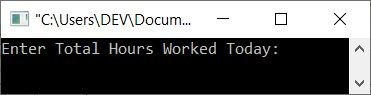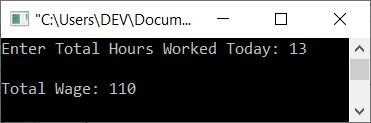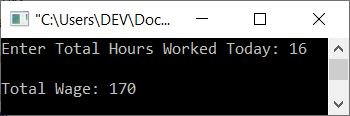# C++ Program for Calculating Labor Wage Based on Hours Worked in a Day

This article provides a program in C++ that calculates and prints the wage of labor based on the hours worked in a day. This program is critical in situations where laborers work by the hour at varying rates.

For example, the program (given below) is created in such a way that the wage of labor must be calculated on the basis of the following rates:

Hours Worked Rate
the first 8 hours 50
next 4 hours 10/hr
next 4 hours 20/hr
next 4 hours 25/hr
next 4 hours 40/hr

## Determine the Labor Wage

The question is: write a program in C++ that calculates and prints the wage of labor based on the total hours worked in a day. The answer to this question is, based on rates as per the table given above:

```#include<iostream>

using namespace std;
int main()
{
float wage=50, hour, th, tw, total_wage;
char name;
cout<<"Enter Total Hours Worked Today: ";
cin>>hour;
if(hour<=8)
total_wage = wage;
else if(hour>8 && hour<=12)
{
th = hour-8;
tw = th*10;
total_wage = tw + wage;
}
else if(hour>12 && hour<=16)
{
th = hour-12;
tw = 4*10;
total_wage = wage + tw + (th*20);
}
else if(hour>16 && hour<=20)
{
th = hour-16;
tw = (4*10) + (4*20);
total_wage = wage + tw + (th*25);
}
else if(hour>20 && hour<=24)
{
th = hour-20;
tw = (4*10) + (4*20) + (4*25);
total_wage = wage + tw + (th*40);
}
else
{
cout<<"\nInvalid Input!";
cout<<"\nHours can't be greater than 24 in a day.";
return 0;
}
cout<<"\nTotal Wage: "<<total_wage;
cout<<endl;
return 0;
}```

Here is the initial output produced by the above program on printing the wage of labor based on hours worked:Now supply the input, say 13, and press the ENTER key to find and print the amount that has to be paid to the labor after working for 13 hours, like shown in the snapshot given below:That is, based on 13 hours, the calculation goes like this:

```13 hours worked = 8 hour + 4 hour + 1 hour
= 50 + (4*10) + (1*20)
= 50 + 40 + 20
= 110```

So for 13 hours, you've paid 110 based on the given rate (in the table). Here's another example with user input:C++ Quiz

« Previous Program Next Program »